## 1. ViT模型介绍

• Transformer相较于CNN结构，缺少一定的平移不变性和局部感知性，因此在数据量不充分时，很难达到同等的效果。具体表现为使用中等规模的ImageNet训练的Transformer会比ResNet在精度上低几个百分点。
• 当有大量的训练样本时，结果则会发生改变。使用大规模数据集进行预训练后，再使用迁移学习的方式应用到其他数据集上，可以达到或超越当前的SOTA水平。

## 2. ViT模型结构与实现

ViT算法的整体结构如 图1 所示。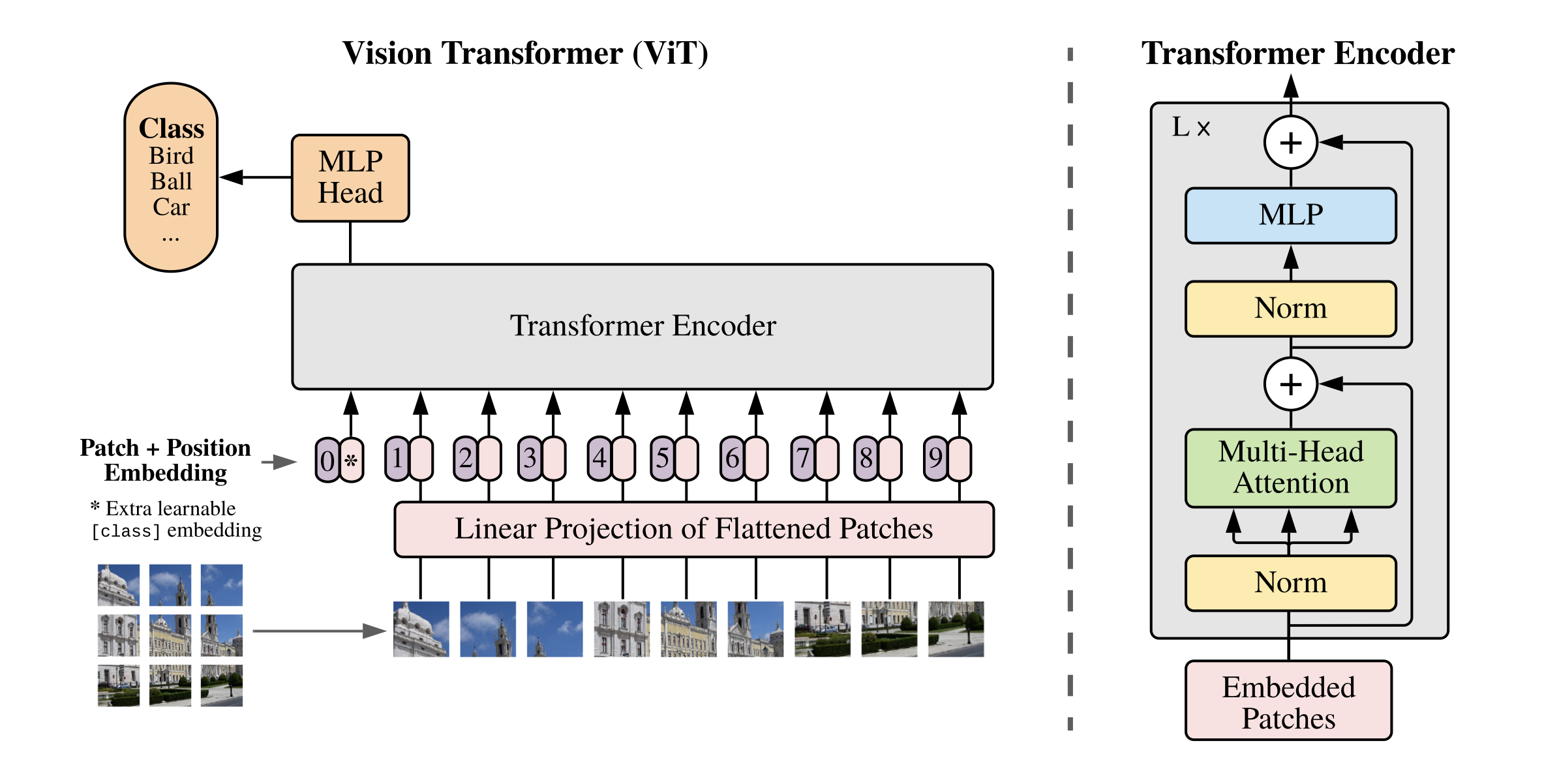### 2.1. 图像分块嵌入

ViT中的具体实现方式为：将 $H \times W \times C$ 的图像，变为一个 $N \times (P^2 * C)$ 的序列。这个序列可以看作是一系列展平的图像块，也就是将图像切分成小块后，再将其展平。该序列中一共包含了 $N=HW/P^2$ 个图像块，每个图像块的维度则是 $(P^2*C)$。其中 $P$ 是图像块的大小，$C$ 是通道数量。经过如上变换，就可以将 $N$ 视为sequence的长度了。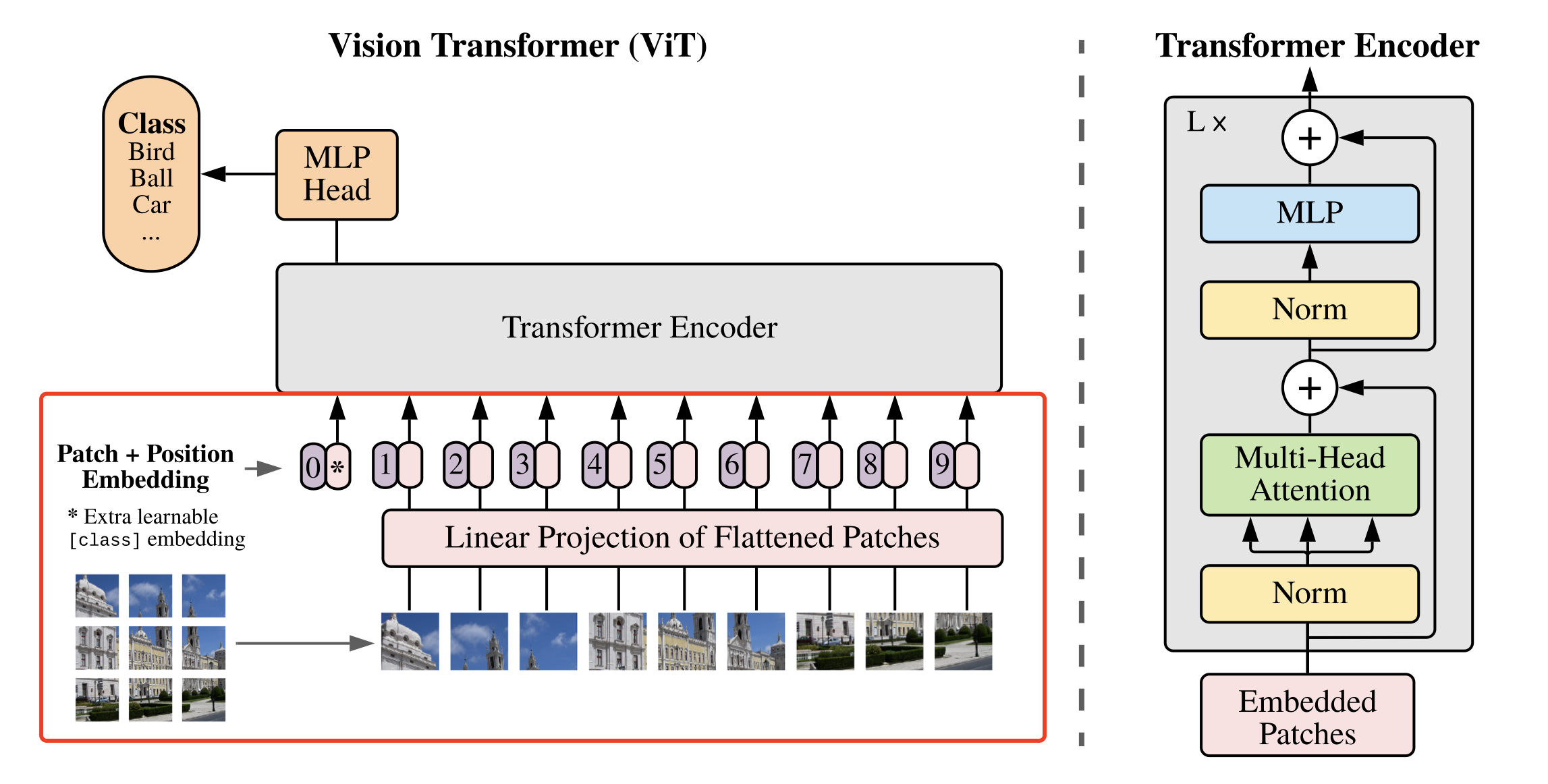# 图像分块、Embedding
class PatchEmbed(nn.Layer):
def __init__(self, img_size=224, patch_size=16, in_chans=3, embed_dim=768):
super().__init__()
# 原始大小为int，转为tuple，即：img_size原始输入224，变换后为[224,224]
img_size = to_2tuple(img_size)
patch_size = to_2tuple(patch_size)
# 图像块的个数
num_patches = (img_size // patch_size) * \
(img_size // patch_size)
self.img_size = img_size
self.patch_size = patch_size
self.num_patches = num_patches
# kernel_size=块大小，即每个块输出一个值，类似每个块展平后使用相同的全连接层进行处理
# 输入维度为3，输出维度为块向量长度
# 与原文中：分块、展平、全连接降维保持一致
# 输出为[B, C, H, W]
self.proj = nn.Conv2D(
in_chans, embed_dim, kernel_size=patch_size, stride=patch_size)

def forward(self, x):
B, C, H, W = x.shape
assert H == self.img_size and W == self.img_size, \
"Input image size ({H}*{W}) doesn't match model ({self.img_size}*{self.img_size})."
# [B, C, H, W] -> [B, C, H*W] ->[B, H*W, C]
x = self.proj(x).flatten(2).transpose((0, 2, 1))
return x

### 2.2. 多头注意力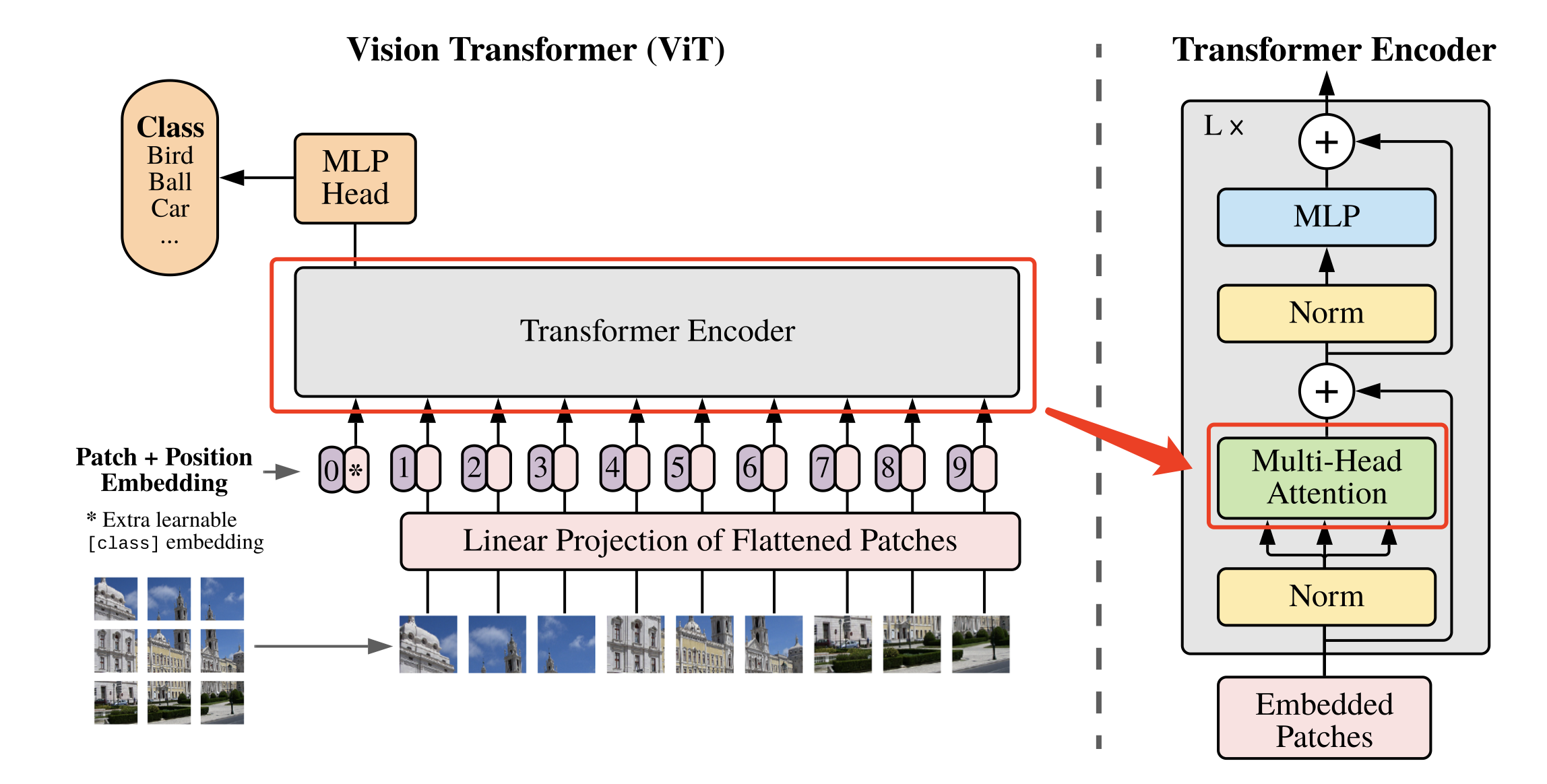Transformer 结构中最重要的结构就是 Multi-head Attention，即多头注意力结构。具有2个head的 Multi-head Attention 结构如 图4 所示。输入 $a^i$ 经过转移矩阵，并切分生成 $q^{(i,1)}$、$q^{(i,2)}$、$k^{(i,1)}$、$k^{(i,2)}$、$v^{(i,1)}$、$v^{(i,2)}$，然后 $q^{(i,1)}$ 与 $k^{(i,1)}$ 做 attention，得到权重向量 $\alpha$，将 $\alpha$ 与 $v^{(i,1)}$ 进行加权求和，得到最终的 $b^{(i,1)}(i=1,2,…,N)$，同理可以得到 $b^{(i,2)}(i=1,2,…,N)$。接着将它们拼接起来，通过一个线性层进行处理，得到最终的结果。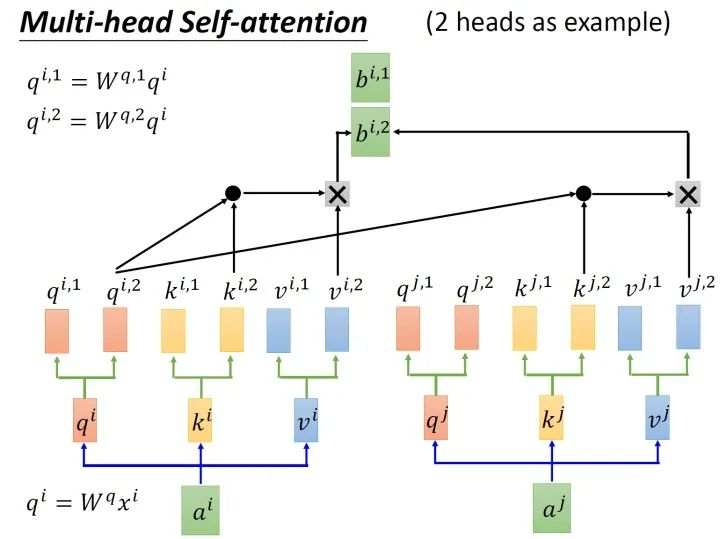$$\alpha_{(1,i)} = q^1 * k^i / \sqrt{d}$$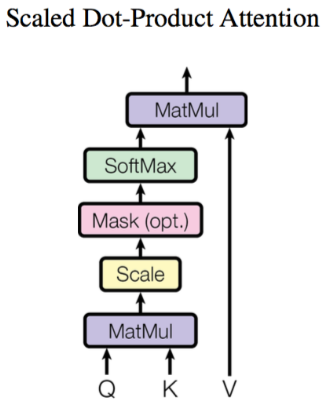# Multi-head Attention
class Attention(nn.Layer):
def __init__(self,
dim,
qkv_bias=False,
qk_scale=None,
attn_drop=0.,
proj_drop=0.):
super().__init__()
# 计算 q,k,v 的转移矩阵
self.qkv = nn.Linear(dim, dim * 3, bias_attr=qkv_bias)
self.attn_drop = nn.Dropout(attn_drop)
# 最终的线性层
self.proj = nn.Linear(dim, dim)
self.proj_drop = nn.Dropout(proj_drop)

def forward(self, x):
N, C = x.shape[1:]
# 线性变换
qkv = self.qkv(x).reshape((-1, N, 3, self.num_heads, C //
# 分割 query key value
q, k, v = qkv, qkv, qkv
# Scaled Dot-Product Attention
# Matmul + Scale
attn = (q.matmul(k.transpose((0, 1, 3, 2)))) * self.scale
# SoftMax
attn = nn.functional.softmax(attn, axis=-1)
attn = self.attn_drop(attn)
# Matmul
x = (attn.matmul(v)).transpose((0, 2, 1, 3)).reshape((-1, N, C))
# 线性变换
x = self.proj(x)
x = self.proj_drop(x)
return x

### 2.3. 多层感知机（MLP）

Transformer 结构中还有一个重要的结构就是 MLP，即多层感知机，如 图6 所示。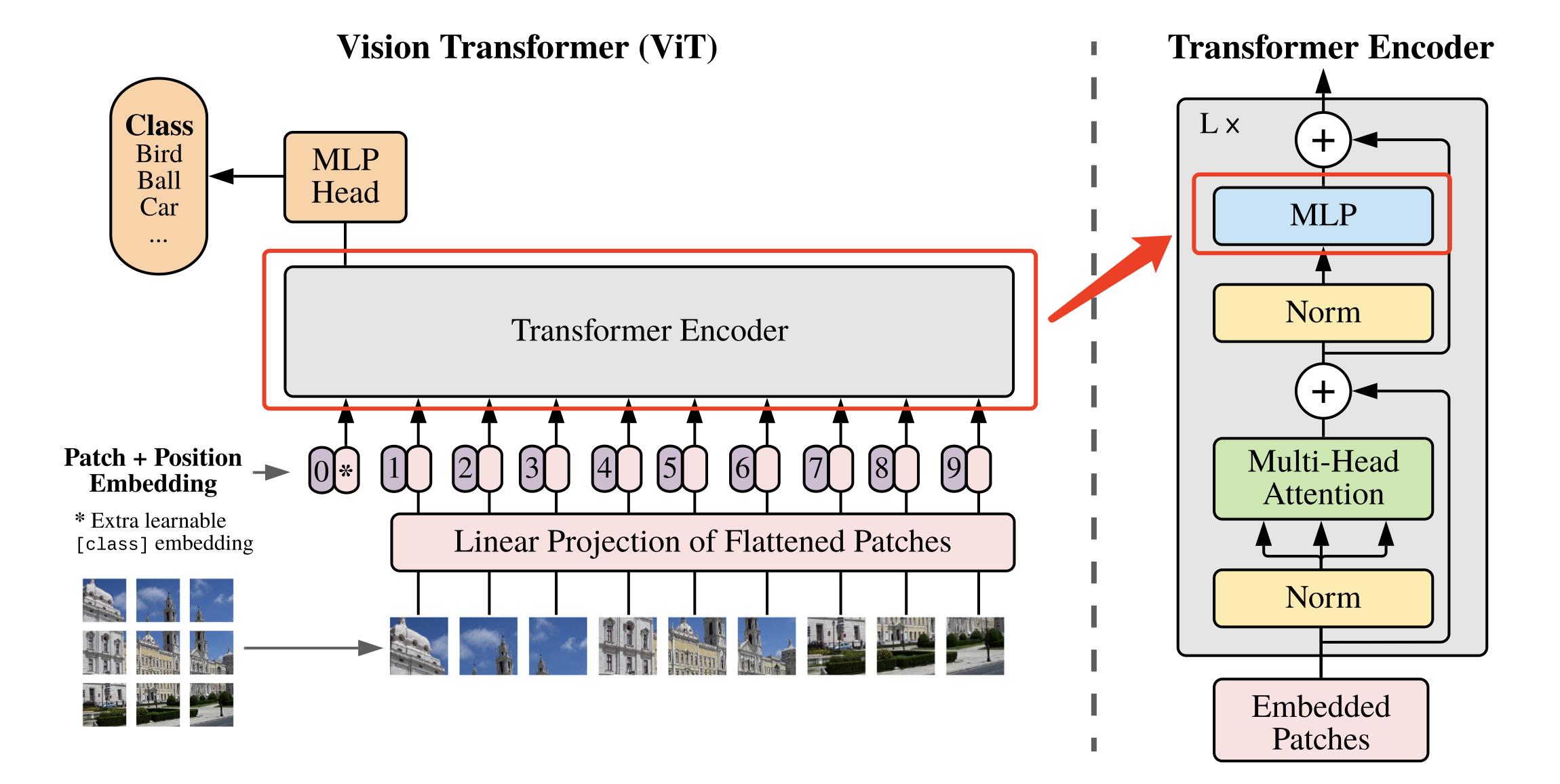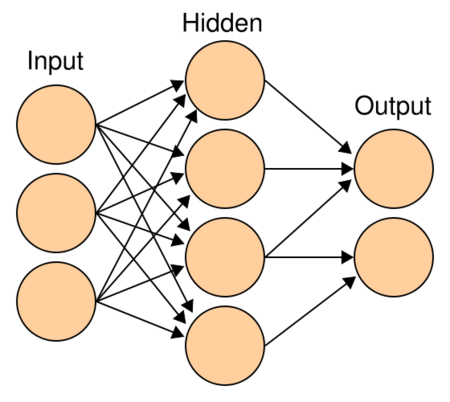class Mlp(nn.Layer):
def __init__(self,
in_features,
hidden_features=None,
out_features=None,
act_layer=nn.GELU,
drop=0.):
super().__init__()
out_features = out_features or in_features
hidden_features = hidden_features or in_features
self.fc1 = nn.Linear(in_features, hidden_features)
self.act = act_layer()
self.fc2 = nn.Linear(hidden_features, out_features)
self.drop = nn.Dropout(drop)

def forward(self, x):
# 输入层：线性变换
x = self.fc1(x)
# 应用激活函数
x = self.act(x)
# Dropout
x = self.drop(x)
# 输出层：线性变换
x = self.fc2(x)
# Dropout
x = self.drop(x)
return x

### 2.4. DropPath

def drop_path(x, drop_prob=0., training=False):
if drop_prob == 0. or not training:
return x
shape = (paddle.shape(x), ) + (1, ) * (x.ndim - 1)
random_tensor = keep_prob + paddle.rand(shape, dtype=x.dtype)
output = x.divide(keep_prob) * random_tensor
return output

class DropPath(nn.Layer):
def __init__(self, drop_prob=None):
super(DropPath, self).__init__()
self.drop_prob = drop_prob

def forward(self, x):
return drop_path(x, self.drop_prob, self.training)

### 2.5. 基础模块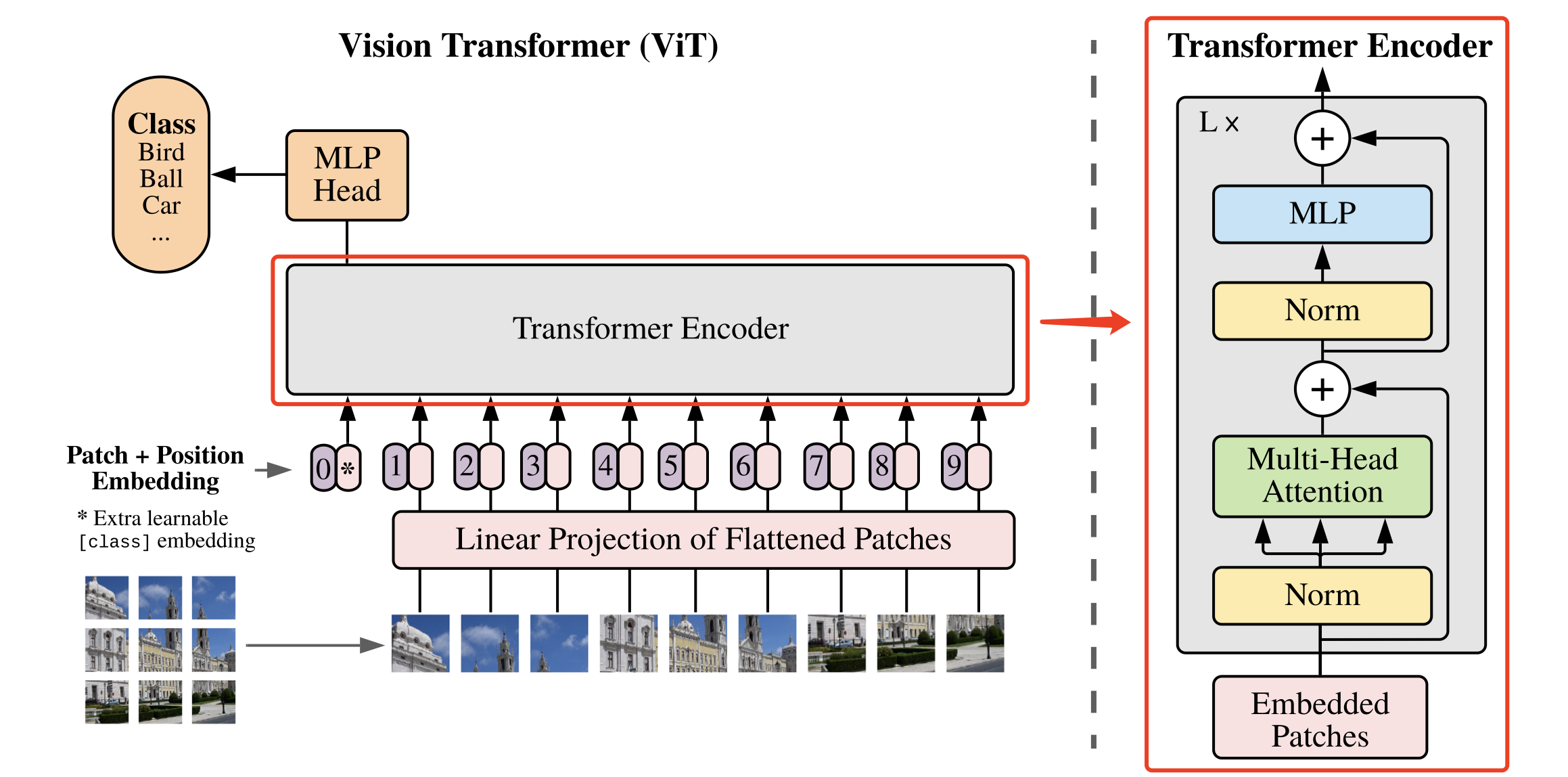class Block(nn.Layer):
def __init__(self,
dim,
mlp_ratio=4.,
qkv_bias=False,
qk_scale=None,
drop=0.,
attn_drop=0.,
drop_path=0.,
act_layer=nn.GELU,
norm_layer='nn.LayerNorm',
epsilon=1e-5):
super().__init__()
self.norm1 = eval(norm_layer)(dim, epsilon=epsilon)
self.attn = Attention(
dim,
qkv_bias=qkv_bias,
qk_scale=qk_scale,
attn_drop=attn_drop,
proj_drop=drop)
# DropPath
self.drop_path = DropPath(drop_path) if drop_path > 0. else Identity()
self.norm2 = eval(norm_layer)(dim, epsilon=epsilon)
mlp_hidden_dim = int(dim * mlp_ratio)
self.mlp = Mlp(in_features=dim,
hidden_features=mlp_hidden_dim,
act_layer=act_layer,
drop=drop)

def forward(self, x):
x = x + self.drop_path(self.attn(self.norm1(x)))
x = x + self.drop_path(self.mlp(self.norm2(x)))
return x

### 2.6. 定义ViT网络

#### 2.6.2. Positional Encoding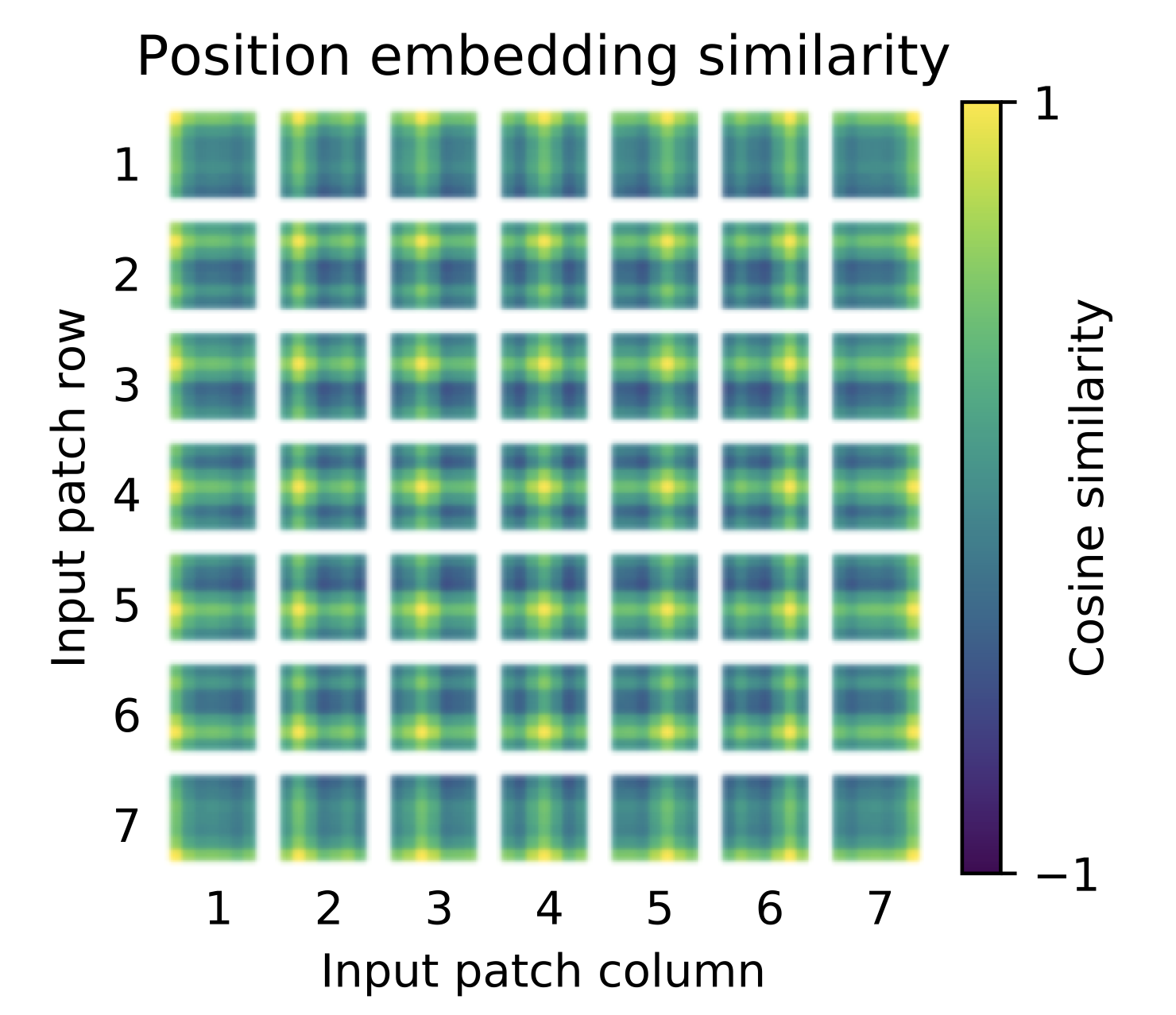# 参数初始化配置
trunc_normal_ = nn.initializer.TruncatedNormal(std=.02)
zeros_ = nn.initializer.Constant(value=0.)
ones_ = nn.initializer.Constant(value=1.)

# 将输入 x 由 int 类型转为 tuple 类型
def to_2tuple(x):
return tuple([x] * 2)

# 定义一个什么操作都不进行的网络层
class Identity(nn.Layer):
def __init__(self):
super(Identity, self).__init__()

def forward(self, input):
return input

class VisionTransformer(nn.Layer):
def __init__(self,
img_size=224,
patch_size=16,
in_chans=3,
class_dim=1000,
embed_dim=768,
depth=12,
mlp_ratio=4,
qkv_bias=False,
qk_scale=None,
drop_rate=0.,
attn_drop_rate=0.,
drop_path_rate=0.,
norm_layer='nn.LayerNorm',
epsilon=1e-5,
**args):
super().__init__()
self.class_dim = class_dim

self.num_features = self.embed_dim = embed_dim
# 图片分块和降维，块大小为patch_size，最终块向量维度为768
self.patch_embed = PatchEmbed(
img_size=img_size,
patch_size=patch_size,
in_chans=in_chans,
embed_dim=embed_dim)
# 分块数量
num_patches = self.patch_embed.num_patches
# 可学习的位置编码
self.pos_embed = self.create_parameter(
shape=(1, num_patches + 1, embed_dim), default_initializer=zeros_)
# 人为追加class token，并使用该向量进行分类预测
self.cls_token = self.create_parameter(
shape=(1, 1, embed_dim), default_initializer=zeros_)
self.pos_drop = nn.Dropout(p=drop_rate)

dpr = np.linspace(0, drop_path_rate, depth)
# transformer
self.blocks = nn.LayerList([
Block(
dim=embed_dim,
mlp_ratio=mlp_ratio,
qkv_bias=qkv_bias,
qk_scale=qk_scale,
drop=drop_rate,
attn_drop=attn_drop_rate,
drop_path=dpr[i],
norm_layer=norm_layer,
epsilon=epsilon) for i in range(depth)
])

self.norm = eval(norm_layer)(embed_dim, epsilon=epsilon)

class_dim) if class_dim > 0 else Identity()

trunc_normal_(self.pos_embed)
trunc_normal_(self.cls_token)
self.apply(self._init_weights)
# 参数初始化
def _init_weights(self, m):
if isinstance(m, nn.Linear):
trunc_normal_(m.weight)
if isinstance(m, nn.Linear) and m.bias is not None:
zeros_(m.bias)
elif isinstance(m, nn.LayerNorm):
zeros_(m.bias)
ones_(m.weight)

def forward_features(self, x):
# 将图片分块，并调整每个块向量的维度
x = self.patch_embed(x)
# 将class token与前面的分块进行拼接
cls_tokens = self.cls_token.expand((B, -1, -1))
# 将编码向量中加入位置编码
x = x + self.pos_embed
x = self.pos_drop(x)
# 堆叠 transformer 结构
for blk in self.blocks:
x = blk(x)
# LayerNorm
x = self.norm(x)
# 提取分类 tokens 的输出
return x[:, 0]

def forward(self, x):
# 获取图像特征
x = self.forward_features(x)
# 图像分类
return x

## 3. 模型指标